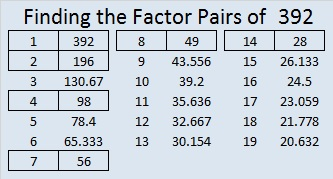# 392 Happy Valentine’s Day

392 is divisible by seven because 39 minus two times the last digit is 35, a multiple of seven. That is one of the reasons 392 is a composite number. All of its factors are listed below the puzzle.Print the puzzles or type the factors on this excel file:12 Factors 2015-02-09

• 392 is a composite number.
• Prime factorization: 392 = 2 x 2 x 2 x 7 x 7, which can be written 392 = (2^3) x (7^2)
• The exponents in the prime factorization are 3 and 2. Adding one to each and multiplying we get (3 + 1)(2 + 1) = 4 x 3 = 12. Therefore 392 has exactly 12 factors.
• Factors of 392: 1, 2, 4, 7, 8, 14, 28, 49, 56, 98, 196, 392
• Factor pairs: 392 = 1 x 392, 2 x 196, 4 x 98, 7 x 56, 8 x 49, or 14 x 28
• Taking the factor pair with the largest square number factor, we get √392 = (√2)(√196) = 14√2 ≈ 19.799This site uses Akismet to reduce spam. Learn how your comment data is processed.